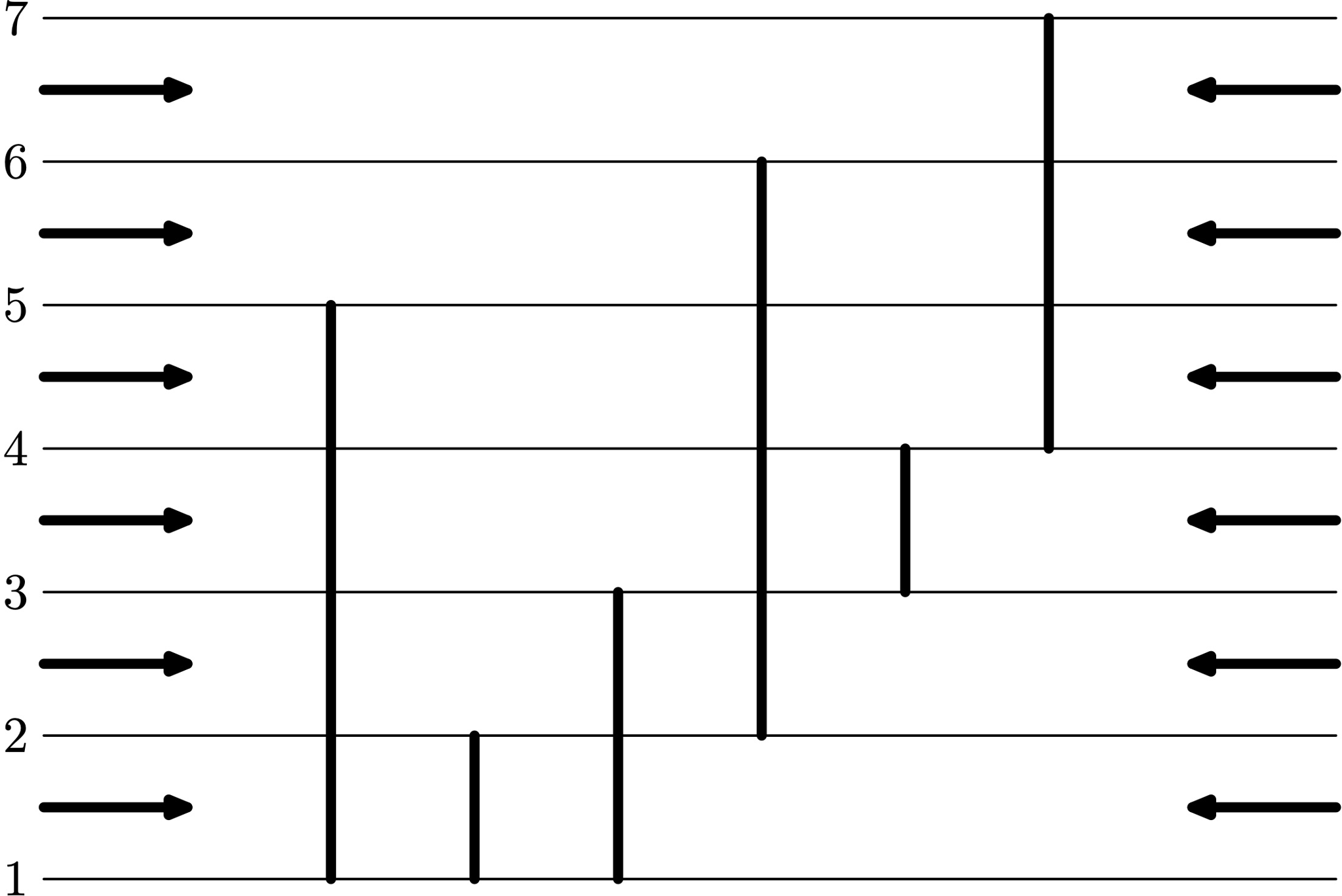시간 제한 메모리 제한 제출 정답 맞은 사람 정답 비율
2 초 256 MB 1 1 1 100.000%

## 문제

Consider a two-dimensional grid with $n$ vertical segments on it. There are two observers, one on the west and one on the east, standing at points on the X axis which are infinitely far from the segments.

Each observer has an x-ray vision of some non-negative integer power that allows him to look through segments. A point of a segment can be seen with vision of power $p$ if there are no more than $p$ other segments crossing the straight line between the observer and this point. We say that a part of a segment is invisible if it is not seen by any of the observers.

You are given $q$ queries. Each query contains two integers: the power of vision of the west and the east observer, respectively. For each query, you need to determine the total length of the invisible parts over all segments.

## 입력

The first line contains one integer $n$ ($1 \le n \le 10^5$), the number of segments.

The $i$-th of the following $n$ lines contains three integers $x_i$, $a_i$, and $b_i$ ($1 \le x \le 10^9$, $1 \le a_i < b_i \le 10^9$), which describe placement of the $i$-th segment: its endpoints have coordinates $(x_i, a_i)$ and $(x_i, b_i)$. It is guaranteed that each segment has positive length and no two segments share a common point.

The next line contains one integer $q$ ($1 \le q \le 10^5$), the number of queries.

Each of the following $q$ lines contains two integers $l$ and $r$ ($0 \le l \le r \le 10^5$), the power of vision of the west and the east observer in this query, respectively.

## 출력

Output $q$ lines, one integer per line: the answers for the corresponding queries.

## 예제 입력 1

6
1 1 5
2 1 2
3 1 3
4 2 6
5 3 4
6 4 7
4
0 0
1 1
0 1
1 0


## 예제 출력 1

4
0
0
0


## 힌트

In the first query, the western observer fully sees the first segment, the part of the fourth segment at Y-coordinates $[5, 6]$, and the part of the sixth one at Y-coordinates $[6, 7]$.

The eastern observer fully sees the fifth and the sixth segments, the part of the fourth segment at Y-coordinates $[2, 3]$, and the part of the third one at Y-coordinates $[1, 2]$.

The parts that remain invisible: the complete second segment, the part of the third one at Y-coordinates $[2, 3]$, and the part of the fourth one at Y-coordinates $[3, 5]$. Their total length is $1 + 1 + 2 = 4$.

In all other queries, there are no invisible parts.